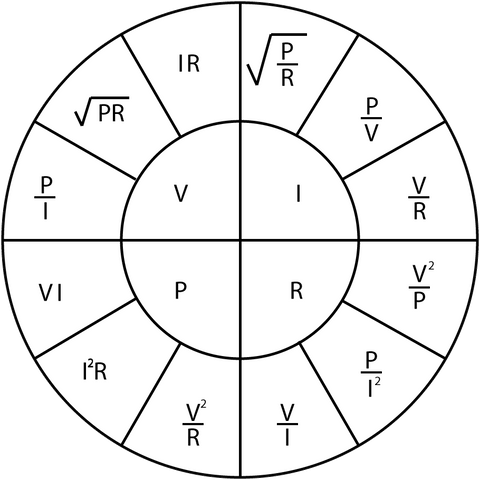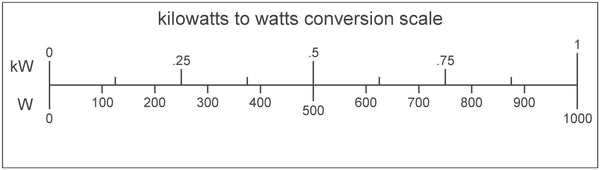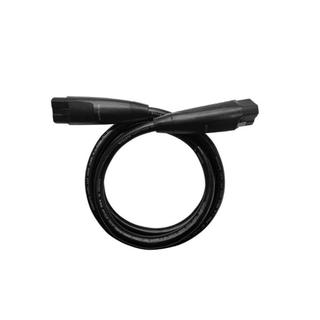Cart30,000+ Happy Customers | Winter Sale on Now! Ends 02/06/23

Need Help? Call:

#####1-877-242-2792

Monday-Friday: 10am-6pm EST
Saturday: 10-4pm EST

# Watt Conversions

By SSK Admin December 09, 2022

Finding the best solar kit for your needs doesn’t have to be a challenge. Using watt conversions, you can calculate your energy requirements and select solar equipment that’ll serve you efficiently.

Usually, these calculations involve having a deep understanding of electrical engineering and other grad school-level concepts. Luckily for you, we’re here to make things a little simpler.

Let’s cover watt conversions in this post to help you understand how to calculate your power requirements and choose the perfect solar equipment.

## What’s a Watt?

Before we get into the calculations, let’s first clarify what a watt is.

A watt, commonly abbreviated as ‘W,’ is the standard unit for measuring power in electrical systems or devices.Power, in this case, is the rate of energy flow in a system. The energy flow occurs in form of currents. That is the movement of charged particles called electrons.

So, watts reveal how fast the electrons within an electrical system move.

The higher the watts, the more energy flows in a circuit. This means that a device with a higher number of watts has more power to perform its intended function than one with low watts.

For instance, a 60 W lightbulb uses three times the current or energy flow to produce light compared to a 20 W bulb everything else being equal.

This means it has more power to produce light and will be brighter.

### Calculating Watts Using Amperes & Volts

To calculate the power of your appliance, two other fundamental units of measurement are necessary – Amperes (A) and Volts (V).

An ampere is a unit that measures current. It gives the specific number of charged particles (electrons) moving through a circuit.

For the electrons to move or flow, a force must push them. The size of this force that sends electrons flowing through a circuit is called volts. Higher voltage in one end of the circuit than another causes the electrons to flow in that direction.

These three units – watts, amperes, and volts – are intricately related. One watt equals one ampere under one voltage.

You can use a Watt’s law calculator to convert one unit to another or follow the following formulas:•  To calculate the number of watts for any electrical system, multiply Amperes and Volts.

P (w) = A x V

For example, A device with 10 Amps and 160 Volts has 1600 watts.

That is, 10 amps * 160 volts = 1600 watts.

•  To work out the number of amps or voltage in a device, given its watts and any of the other two fundamental electrical units, divide watts by volts or Amps
• Finding Amps = Watts / Volts
• Finding Volts = Watts / Amps

Let’s take one of our small flexible solar panels as an example - the Renogy 100 Watt, 12 V Flexible Monocrystalline Solar Panel.

To find amps take 100 watts / 12 volts = 8.3 Amps

But if we had the value of watts (100) and amps (8.3) instead, we'd calculate voltage as

100 watts / 8.3 amps to get the 12 volts.## Watt Conversions

There are different categories of measuring power depending on the capacity of an electric system or device.

Microwatts (µW) and milliwatts (mW) measure the power in devices with a relatively small capacity, like hearing aids.

On the other hand, kilowatts (kW) and megawatts (MW) measure the power in systems with a high capacity, such as engines, motors, power plants, etc.

You can convert watts into any of the other units of measurement.

Here are the formulas you need to convert watts to:

• Microwatts – Multiply the number of watts by 1,000,000
• Milliwatts – Multiply watts by 1000
• Kilowatts – Divide watts by 1000
• Megawatts – Divide watts by 1,000,000

You can also convert watts to watt hours to calculate your power requirements.

A watt-hour (Wh) measures a device’s energy usage over a specific time, usually one hour.

To calculate watt-hours, use the formula – Watts times hours.Example:

To find the daily watt-hour consumption for a 1,100-watt electric kettle used 30 minutes a day, you’d take the:

1,100 W x 0.5 (hours in a day) = 550 watt-hours.

Since the most common metric used to measure energy consumption is the kilowatt hours (kWh), convert the watt hours into kilowatt hours – divide watt-hours by 1000.

550 / 1000 = 0.55 kWh

So, to run the electric kettle, you’ll need a solar system than can produce around 0.55 kWh or more.

Finding such a system involves calculating a battery’s watt-hours.

### Calculating the Battery Capacity You Need

Most batteries display the amp-hours and volts requirements as part of their description.

Amp-hours (Ah) is the standard unit that measures a battery’s capacity. It gives the amount of charge stored in a battery.  However, it’s not always an accurate estimate of how much energy a specific battery can deliver.

That’s because the total amount of energy a battery provides relies on its amp hours (Ah) and voltage (V) since batteries change voltage when discharging.

For a more accurate estimate of the battery capacity sufficient to meet your needs, convert amp hours to watt-hours. How? By multiplying amp hours and voltage.

For instance, if you have a 12 V 60 Ah battery, you can calculate its capacity by taking the:

60 Amp hours (Ah) times 12 Volts (V) to get 1,500 Watt hours (Wh)

To convert the watt hours into kilowatt hours – divide the watt hours by 1,000

1,500 / 1,000 = 1.5 kWh.

Such a battery would, therefore, power the electric kettle explained above efficiently for a few days.You can also convert watt hours to amp hours.  All you have to do is divide the number of watt-hours by volts.

Amp-hours (Ah) equals Watt hours (Wh) / Volts (V)

If you’d like to convert watt-hours into milliamp hours instead, you’ll first have to convert watt-hours into amp hours, as shown above, and multiply by 1,000.

## Conclusion

Now that you know how to calculate your power needs and battery capacity, you can always find a solar kit to match your needs.

You’ll have to identify the daily consumption rates for all the devices you wish to run with your solar equipment. But this shouldn’t worry you since there are several calculators online, including a watts-to-amp hour calculator, to help you out.

Did You Find Our Blog Helpful? Then Consider Checking:

### Recent Post

•### Bluetti AC200P vs Ecoflow Delta 1800: Which is Best?

Jan 11, 2023
•### Convert Amps to Watts

Jan 07, 2023
•### Amp-Hour Calculator

Jan 07, 2023
•### Amp-Hours to Watts Calculator

Jan 07, 2023
•### Amps to Volts Calculator

Jan 07, 2023
•### Amps, Watts, & Volts Calculator

Jan 07, 2023
•### Amps to kW Calculator

Jan 07, 2023
•### Amp Wire Gauge Calculator

Jan 07, 2023
•### Amp Draw Calculator

Jan 07, 2023
•### Amps to VA Calculator

Jan 07, 2023

### CategoriesSomeone purchased a

#### EcoFlow Delta 1800W / 1,300wH [Quad Kit] +...

59 minutes agoSomeone purchased a

#### Complete Off-Grid Solar Kit - MPP LV2424 2,400W...

44 minutes agoSomeone purchased a

#### Complete Off-Grid Solar Kit - 4,000W 120/240V Output...

58 minutes agoSomeone purchased a

#### EcoFlow DELTA PRO 3,600wH / 3,600W [Hex Kit]...

49 minutes agoSomeone purchased a

#### Complete Off-Grid Solar Kit - 6,000W 120/240V Output...

21 minutes agoSomeone purchased a

#### Complete Off-Grid Solar Kit - 6,000W 120/240V Output...

54 minutes agoSomeone purchased a

#### Complete Off-Grid Solar Kit - 6,000W 120/240V Output...

32 minutes agoSomeone purchased a

#### EcoFlow DELTA [PRO] 3,600wH / 3,600W [NOMAD Kit]...

59 minutes agoSomeone purchased a

#### Complete DIY Solar Panel Kit - 3,000W Inverter...

34 minutes agoSomeone purchased a

#### EcoFlow Smart Home Panel Extra Infinity Cable

46 minutes agoSomeone purchased a

#### Complete Off-Grid Solar Kit - 6,000W 120/240V Output...

58 minutes agoSomeone purchased a

#### Complete Off-Grid Solar Kit - 6,000W 120/240 Output...

43 minutes agoSomeone purchased a

#### Complete Solar Kit - 7,200W 120/240V Output /...

37 minutes agoSomeone purchased a

#### Complete DIY Solar Panel Kit - 2,000W Pure...

29 minutes agoSomeone purchased a

#### EcoFlow DELTA PRO 3,600wH / 3,600W [Quad Kit]...

56 minutes agoSomeone purchased a

#### Complete Hybrid Solar Kit - Sol-Ark 12K 120/240V...

31 minutes agoSomeone purchased a

#### Complete Off-Grid Solar Kit - 13,000W 120/240V Output...

31 minutes agoSomeone purchased a

#### Complete Off-Grid Solar Kit - 13,000W 120/240V +...

50 minutes ago